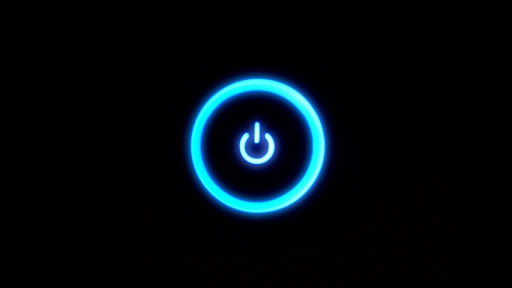Open in App
Not now

# Python – Remove Part of an Image

• Last Updated : 17 Dec, 2020

Removing part of an image refers to the process of destroying image data in certain regions of an image. Where the removal can be either dynamic or hard-coded. In most removal processes the dimensions of the image stay the same in the resultant image. Literally removing sections from an image would change the dimensions in unaccountable ways. In this article, we will take a look at ways of removing a region of an image, and would learn about different ways of doing so.

## Why remove a region from an image?

Sometimes an image contains certain artifacts (irregularities) or unwanted regions in it that may be undesirable. Once such regions have been recognized then identifying their types is necessary, which would help in coming up with the best method to get rid of them. Most Image processing packages like Photoshop, Gimp etc. do offer tools for performing the specific task. But this can also be done programmatically as we will see in a moment. The following image will be used for demonstration.Example 1:

Removing a part (Region) from an image requires the Region of interest to be provided beforehand. Where providing ROI each time the process is undergone means the ROI is hardcoded. Whereas computation of ROI by itself (changing accordingly with different image and conditions) means the ROI is dynamic. Where the ROI is generally a size 4 tuple which contains the top left and bottom right coordinates of the Bbox.

Removing a region means we first select the region we are willing to get rid of. Where the selection could be Region or Pixel value-based. Once the region has been identified we will turn the pixel values of that region to the ones of the background. Where background color is not constant, and hence depends upon the context for which the image is to be used. The most common backgrounds are either white or black. In this article, we will assume that the background color is black. To demonstrate this we would be removing the pixels in the region (0,0) to (400, 400) (top left side) of the aforementioned image.

Below is the implementation:

## Python3

 `from` `PIL ``import` `Image``import` `numpy as np      `` ` `# Opening the image and converting ``# it to RGB color mode``# IMAGE_PATH => Path to the image``img ``=` `Image.``open``(r``"IMAGE_PATH"``).convert(``'RGB'``)`` ` `# Extracting the image data &``# creating an numpy array out of it``img_arr ``=` `np.array(img)`` ` `# Turning the pixel values of the 400x400 pixels to black ``img_arr[``0` `: ``400``, ``0` `: ``400``] ``=` `(``0``, ``0``, ``0``)`` ` `# Creating an image out of the previously modified array``img ``=` `Image.fromarray(img_arr)`` ` `# Displaying the image``img.show()`

Output:Top left region of the image removed

Explanation:

Firstly we imported the PIL image library and Numpy module which would allow us to store homogeneous pixel values as arrays which in turn leads to faster operations on them. Then we opened the image (created image object) using Image.open() function and later converted the image to RGB color mode (originally it was RGBA). After which we created a Numpy array out of the image data using np.array() function. Later we utilized indexed slicing to turn the pixel values of region (0, 0) – (400, 400) to black (0, 0, 0). In the end, we created an image from the modified pixel data using Image.fromarray() and displayed it.

Example 2: Removing region of an image having rgba color mode

If an image is of RGBA color mode, then the removed region need not be represented using a color value (as in the previous case). We could make the removed regions appear as completely transparent (having alpha 0). This not only serves the purpose of removing that region from the final image (or sort of disappearing) but also gives a visual cue of nonexistent pixels in that region. To demonstrate this the following image will be used:-We would be removing the regions of the image which are filled with black color. Since the region could not be described as a shape, we would be using floodfill functions to fill that region with transparent pixel values. In the following example, we would be using the magenta color value as a seed to the floodfill algorithm, and would use that to get fully transparent pixel values.

Below is the implementation:

## Python3

 `# Importing ImageDraw for``# using floodfill function``from` `PIL ``import` `Image, ImageDraw`` ` `# Opening the image and``# converting its type to RGBA``img ``=` `Image.``open``(r``"IMG_PATH"``).convert(``'RGBA'``)`` ` `# Location of seed``seed ``=` `(``0``, ``0``)`` ` `# Pixel Value which would``# be used for replacement``rep_value ``=` `(``0``, ``0``, ``0``, ``0``)`` ` `# Calling the floodfill() function and``# passing it image, seed, value and``# thresh as arguments``ImageDraw.floodfill(img, seed, rep_value, thresh ``=` `100``)`` ` `img.show()`

Output:It may appear as if the black color in the image got replaced by white color. But that white color is in fact the color of the background, the pixels of that regions are fully transparent (having alpha = 0). What we did in the above code is:

1. Firstly found out the longest continuous region consisting of black color (using flood/seed fill algorithm to do so)
2. Change the color values of that region to (0, 0, 0, 0) (full transparency)

My Personal Notes arrow_drop_up